### Home > CALC > Chapter Ch3 > Lesson 3.2.2 > Problem3-66

3-66.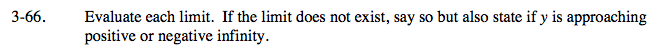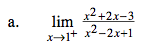The denominator equals zero. Factor first to see if you can cancel out that zero. If so, the limit exists. If not, it DNE but approaches +∞ or −∞.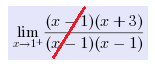Even though some cancelling happens, there is still an
(x − 1) on the bottom. That means that the limit DNE but
y → ∞ or y → − ∞. Which one?

For more guidance, refer to the hint in problem 3-51(a).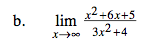The highest-power term in the numerator is x2.
The highest-power term in the denominator is 3x2.
When x→∞, only the highest-power terms need to be considered.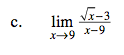There appears to be a zero in the denominator. But can you factor and cancel?

Or you could multiply the numerator and denominator by the conjugate of the numerator.

Or you might have recognized that this is Ana's Definition of the Derivative:

$f'(a)=\lim_{x\rightarrow a}\frac{f(x)-f(a)}{x-a}$

$\text{Examining closely, }a=9 \text{ and }f(x)=\sqrt{x}. \text{ Find }f'(x) \text{ and, finally, }f'(a).$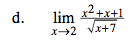Sometimes, a limit can be evaluated in just one step. In a case like this, the value of the limit is the same as the real value (there are no holes on this graph).